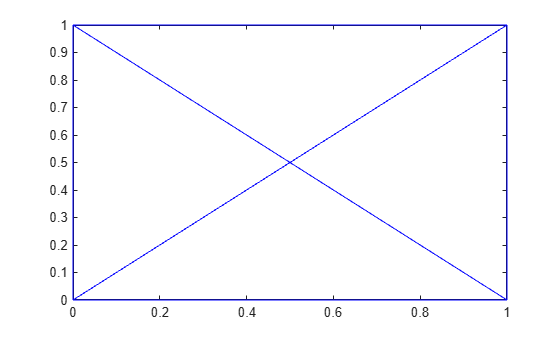# edgeAttachments

Triangles or tetrahedra attached to specified edge

## Syntax

``ID = edgeAttachments(TR,startID,endID)``
``ID = edgeAttachments(TR,E)``

## Description

example

````ID = edgeAttachments(TR,startID,endID)` identifies the triangles or tetrahedra attached to the specified edges. `startID` and `endID` specify edges by their start and end points, where each point is represented by its row number in `TR.Points`. The return value `ID` identifies triangles by their identification numbers.```

example

````ID = edgeAttachments(TR,E)` specifies the starting and ending vertices of each edge in a two-column matrix `E`.```

## Examples

collapse all

Create and plot a Delaunay triangulation.

```x = [0 1 1 0 0.5]'; y = [0 0 1 1 0.5]'; TR = delaunayTriangulation(x,y); triplot(TR)```Compute the identifications of the triangles attached to the edge defined by the starting point (0,0) and ending point (0.5,0.5). The respective vertex identifications of these points are 1 and 5.

```ID = edgeAttachments(TR,1,5); ID{:}```
```ans = 1×2 4 1 ```

The `ConnectivityList` property indicates which vertices belong to each triangle. The fourth triangle is defined by vertices (0.5,0.5), (0,0), and (1,0), and the first triangle is defined by vertices (0,1), (0,0), and (0.5,0.5).

`TR.ConnectivityList`
```ans = 4×3 4 1 5 4 5 3 5 2 3 5 1 2 ```
`TR.Points`
```ans = 5×2 0 0 1.0000 0 1.0000 1.0000 0 1.0000 0.5000 0.5000 ```

Load 2-D triangulation data and create a triangulation representation.

```load tetmesh TR = triangulation(tet,X);```

Select two edges by their starting and ending vertex identifications.

```startID = [15; 21]; endID = [936; 716];```

Find the edge attachments, and examine the identifications of the triangles attached to each edge.

```ID = edgeAttachments(TR,startID,endID); ID{1}```
```ans = 1×6 927 2060 3438 3423 2583 4690 ```
`ID{2}`
```ans = 1×5 2652 3946 3953 4665 4218 ```

## Input Arguments

collapse all

Triangulation representation, specified as a scalar `triangulation` or `delaunayTriangulation` object.

Data Types: `triangulation` | `delaunayTriangulation`

Starting vertex identification for each edge, specified as a column vector. A vertex identification number is the number of the row in the `Points` property that corresponds to the vertex.

Data Types: `double`

Ending vertex identification for each edge, specified as a column vector. A vertex identification number is the number of the row in the `Points` property that corresponds to the vertex.

Data Types: `double`

Edge matrix, specified as a two-column matrix. Each row contains the starting and ending vertex identifications for an edge. A vertex identification number is the number of the row in the `Points` property that corresponds to the vertex.

Data Types: `double`